Question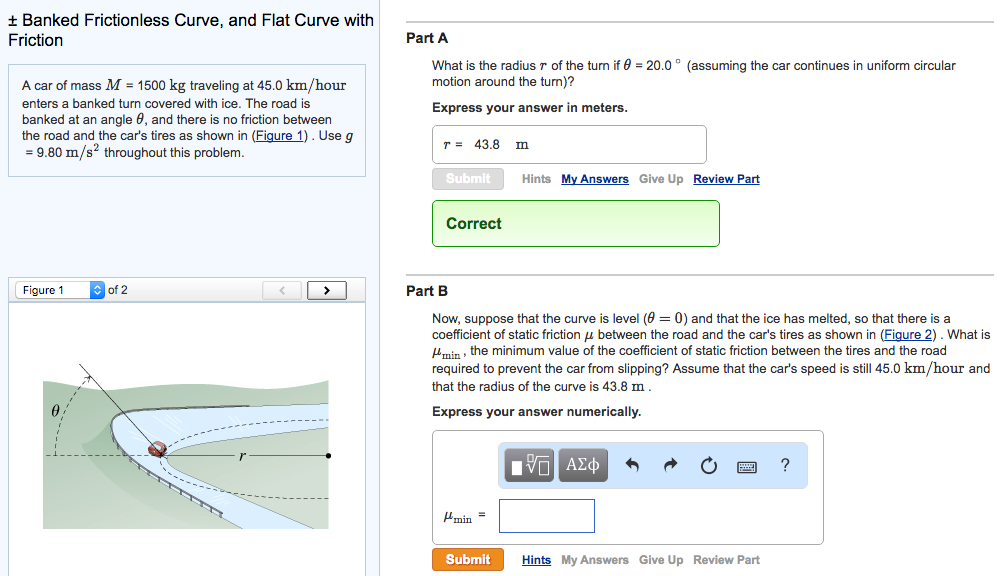PLEASE ANSWER PART B. THANKS!

if theta = 0 degrees

then v^2 = mu*rg

coefficient of static friction is mu_min = v^2/(r*g)

v = 45 km/hr = 45*5/18 = 12.5 m/sec

mu_min = 12.5^2/(43.8*9.8) = 0.364

#### Earn Coins

Coins can be redeemed for fabulous gifts.

Similar Homework Help Questions
• ### Banked Frictionless Curve, and Flat Curve with Friction Reviev A car of mass M - 1300...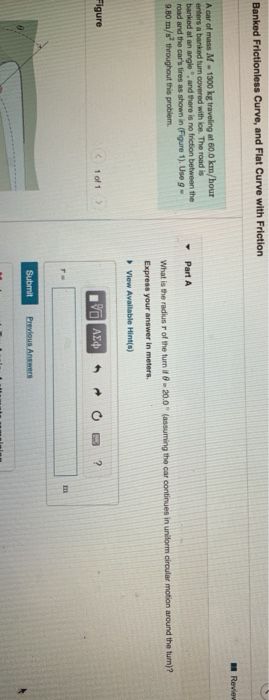Banked Frictionless Curve, and Flat Curve with Friction Reviev A car of mass M - 1300 kg traveling at 60.0 km/hour enfers a banked turn covered with ice. The road is banked at an angle and there is no friction between the road and the car's tires as shown in Figure 1). Use 9 - 9.B0 m/s throughout this problem. Part A What is the radius of the turn it 8 - 20.0 (assuming the car continues in uniform circular...

• ### Banked Frictionless Curve, and Flat Curve with Friction 10 of 19 > Constants • En a...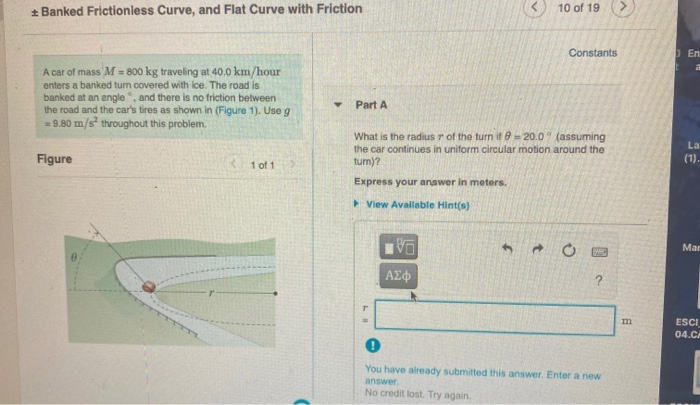Banked Frictionless Curve, and Flat Curve with Friction 10 of 19 > Constants • En a A car of mass M = 800 kg traveling at 40.0 km/hour enters a banked turn covered with ice. The road is banked at an angle, and there is no friction between the road and the car's tires as shown in (Figure 1). Use = 9.80 m/s throughout this problem. Part A What is the radius r of the turn it e = 20.0°...

• ### A car of mass M = 800 kg traveling at 55.0 km/hour enters a banked turn...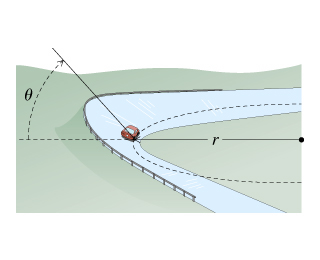A car of mass M = 800 kg traveling at 55.0 km/hour enters a banked turn covered with ice. The road is banked at an angle ?, and there is no friction between the road and the car's tires as shown in(Figure 1) . Use g = 9.80 m/s2 throughout this problem. Now, suppose that the curve is level (?=0) and that the ice has melted, so that there is a coefficient of static friction ? between the road and...

• ### A car of mass M = 1300 kg traveling at 65.0 km/hour enters a banked turn...

A car of mass M = 1300 kg traveling at 65.0 km/hour enters a banked turn covered with ice. The road is banked at an angle θ, and there is no friction between the road and the car's tires as shown in (Figure 1) . Use g = 9.80 m/s2 throughout this problem. r= 91.43 m. Now, suppose that the curve is level (θ=0) and that the ice has melted, so that there is a coefficient of static friction μ...

• ### Now, suppose that the curve is level (θ=0) and that the ice has melted, so that...

Now, suppose that the curve is level (θ=0) and that the ice has melted, so that there is a coefficient of static friction μ between the road and the car's tires as shown in (Figure 2). What is μmin, the minimum value of the coefficient of static friction between the tires and the road required to prevent the car from slipping? Assume that the car's speed is still 45.0 km/hour and that the radius of the curve is 43.8 m.

• ### Now, suppose that the curve is level (θ=0 ) and that the ice has melted, so...

Now, suppose that the curve is level (θ=0 ) and that the ice has melted, so that there is a coefficient of static friction μ between the road and the car's tires. What is μ min   , the minimum value of the coefficient of static friction between the tires and the road required to prevent the car from slipping? Assume that the car's speed is still 45.0 km/hour and that the radius of the curve is 43.8 m .

• ### A car of mass M= 1300 kg traveling at 65.0 km/hour enters a banked turn covered with ice. The road is banked at an ang...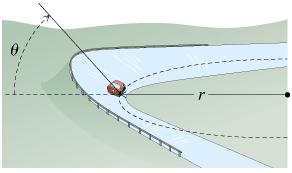A car of mass M= 1300 kg traveling at 65.0 km/hour enters a banked turn covered with ice. The road is banked at an angle , and there is no friction between the road and the car's tires. (Intro 1 figure) . Use g= 9.80 m/s^2 throughout this problem. What is the radius (in meters) of the turn if = 20.0 (assuming the car continues in uniform circular motion around the turn)?

• ### A car of mass M = 1500 kg traveling at 65.0 km/hour enters a banked turn...

A car of mass M = 1500 kg traveling at 65.0 km/hour enters a banked turn covered with ice. The road is banked at an angle ∘, and there is no friction between the road and the car's tires as shown in (Figure 1). Use g = 9.80 m/s2 throughout this problem. What is the radius r of the turn if θ = 20.0 ∘ (assuming the car continues in uniform circular motion around the turn)?

• ### A car of mass M = 1300 kg traveling at 45.0 km/hour enters abanked turn...

A car of mass M = 1300 kg traveling at 45.0 km/hour enters a banked turn covered with ice. The road is banked at an angle θ, and there is no friction between the road and the car's tires as shown in (Figure 1) . Use g = 9.80 m/s2 throughout this problem. What is the radius r of the turn if θ = 20.0 ∘ (assuming the car continues in uniform circular motion around the turn)?

• ### A car of mass M = 1400 kg traveling at 65.0 km/hour enters a banked turn covered with ice. The...

Now, suppose that the curve is level (theta = 0) and that the ice has melted, so that there is a coefficient of static friction mu between the road and the car'stires. (Part B figure) What is mu_min, the minimum value of the coefficient of static friction between the tires and the road required to prevent the car fromslipping? Assume that the car's speed is still 65.0 km/hour and that the radius of the curve is given by the value...